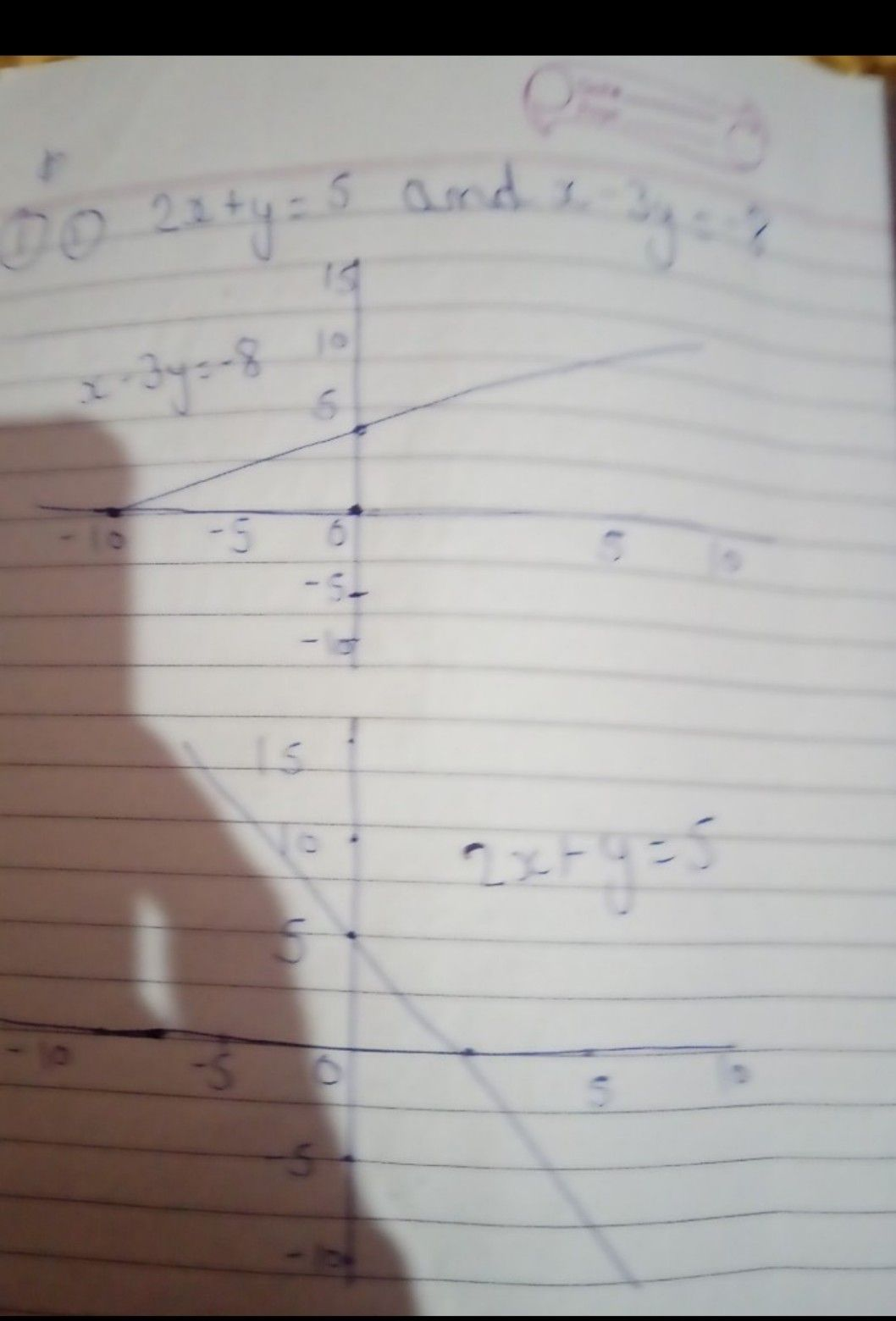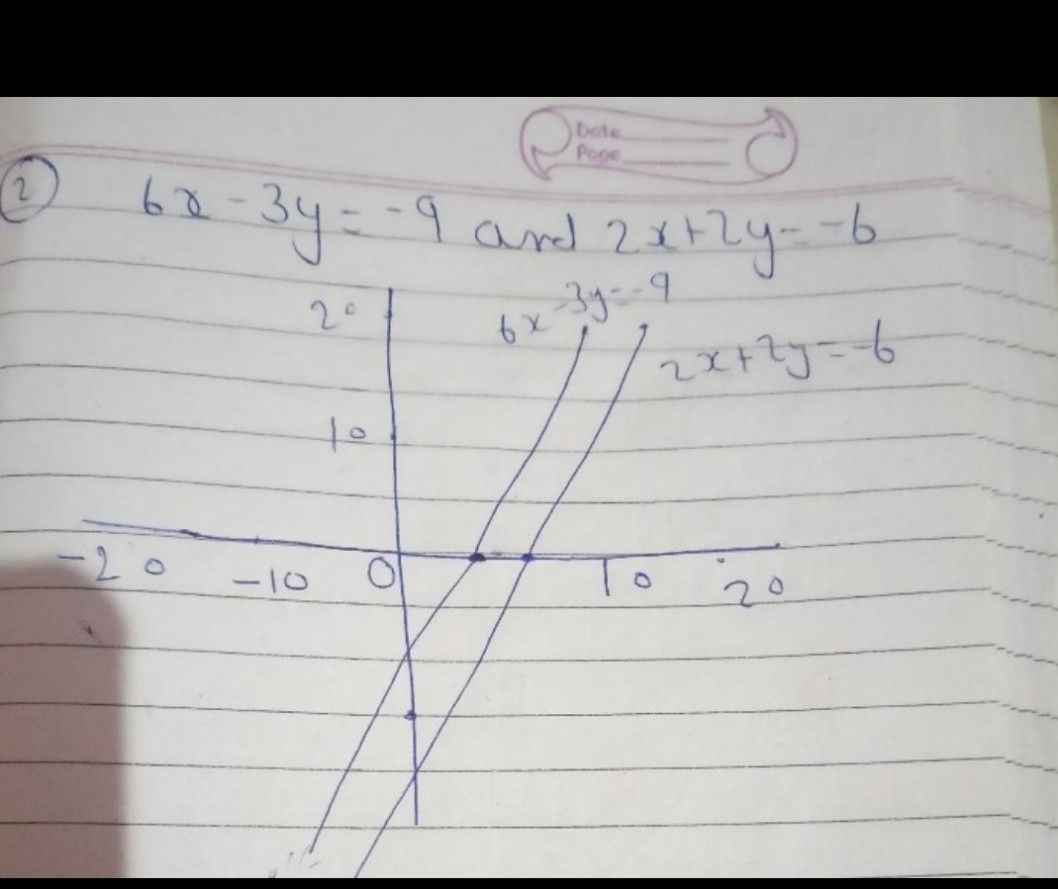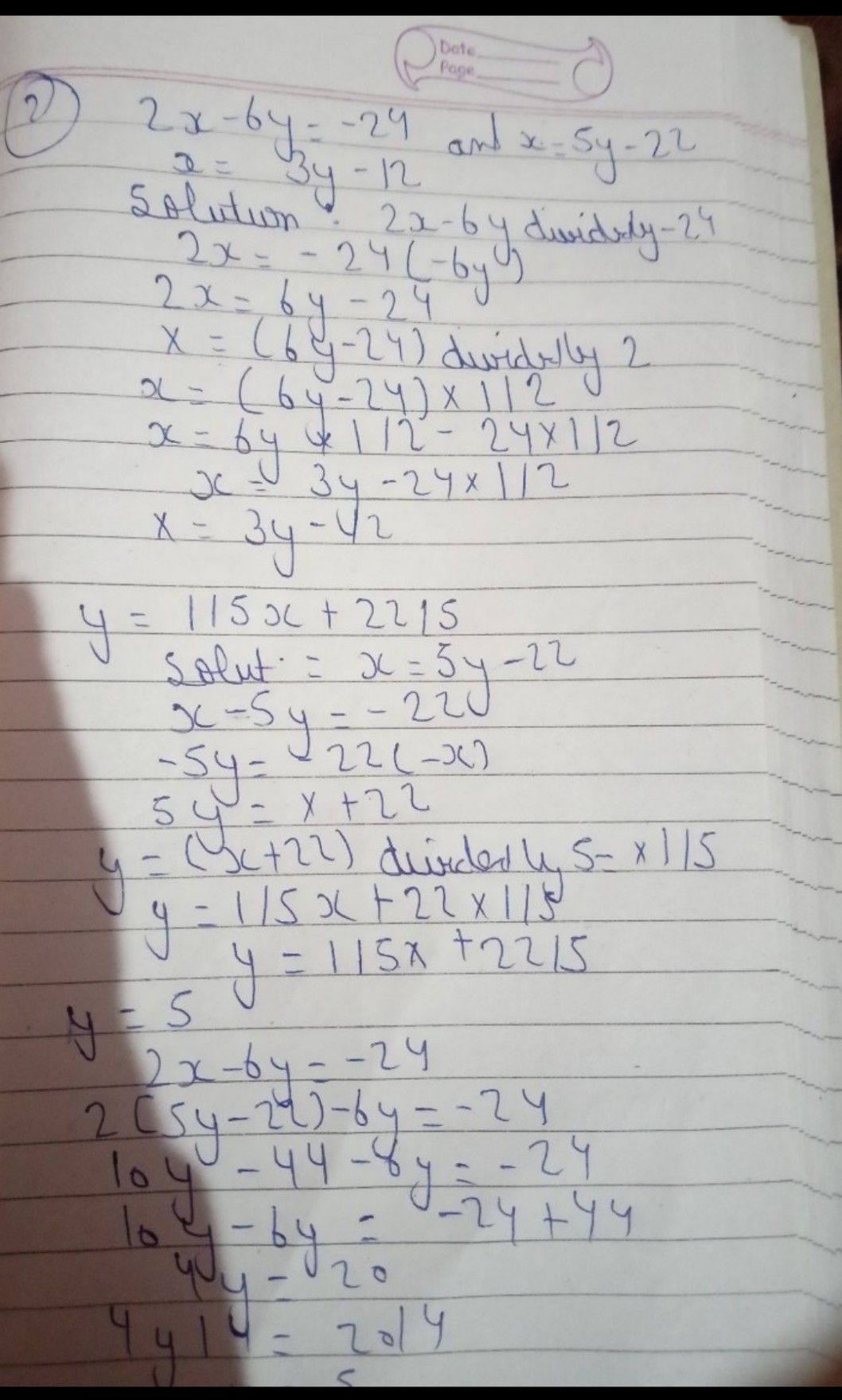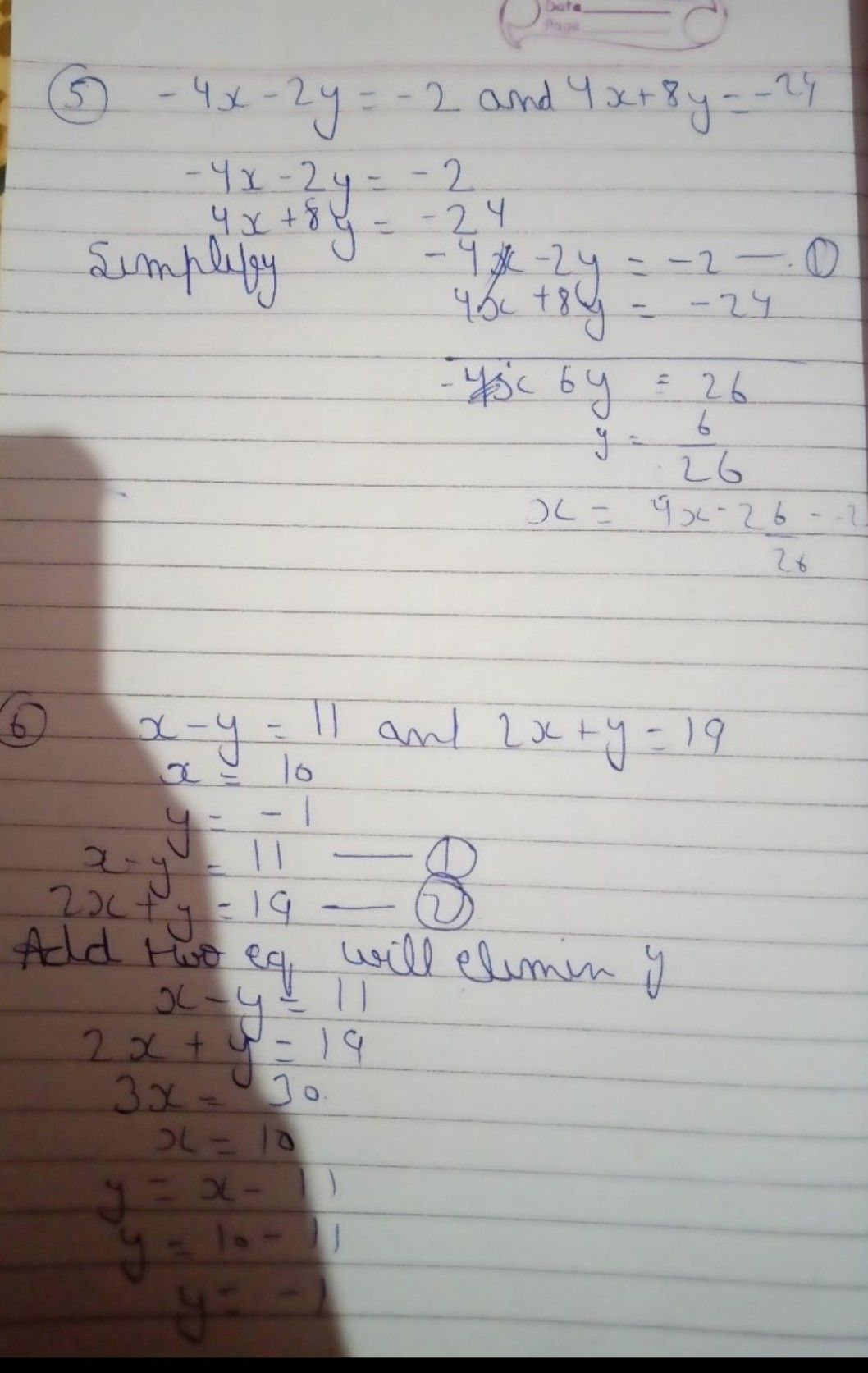Symbol
ProblemSolves a systems of linear equations in two variables. Solve the following systems by graphing. $1\right)$ $2x+y=5$ and $x-3y=-8$ $2\right)$ $6x-3y=-9$ and $2x+2y=-6$ Solve the following systems by substitution $3\right)$ $y=5x-3$ and $-x-5y=-11$ $4\right)$ $2x-6y=-24$ and $x=5y-22$ Solve the following systems by elimination $5\right)$ $-4x-2y=-2$ and $4x+8y=-24$ $6\right)$ $x-y=11$ and $2x+y=19$
7th-9th grade
Other
Search count: 124
SolutionQanda teacher - light